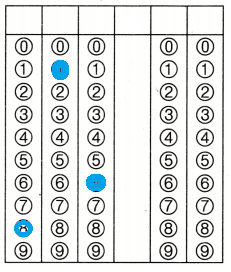# Texas Go Math Grade 4 Module 8 Assessment Answer Key

Refer to our Texas Go Math Grade 4 Answer Key Pdf to score good marks in the exams. Test yourself by practicing the problems from Texas Go Math Grade 4 Module 8 Assessment Answer Key.

## Texas Go Math Grade 4 Module 8 Assessment Answer Key

Vocabulary

• counting number5
• compatible numbers
• products

Vocabulary

Choose the best term from the box to complete the sentence.

Question 1.
_______________ are numbers that are easy to compute mentally.
Compatible numbers
Explanation:
The numbers which are close to the actual numbers, and can be easily added, subtracted or divided orally are known as compatible numbers.”. These numbers are used for carrying out basic mathematical functions.
For example;
Compatible numbers for 232 and 11 are 240 and 12.

Concepts and Skills

Question 2.
Explain how to find 4 × 50 using mental math.
4 x 50
4 x 5 x 10
20 x 10 = 200
Explanation:
Multiplying by 10 and 100 Using a Place-Value-Change Strategy
This strategy involves keeping track of how the place values change when a number is multiplied by 10.

Question 3.
What is the first step in estimating 56 × 27?
56 is rounded to 60 and 27 id rounded to 30
60 x 30 = 1800
56 x 27 = 1512
Explanation:
Observing the first place of a given numbers,
Estimates can be very broad and general or
they can be quite close to the actual answer.
It all depends on the reason for estimating in the first place, then second tenth place digit.

Choose a method. Then find the product.

Question 4.
35 × 10 ___________
Explanation:
30 x 10 + 5 x 10
300 + 50 = 350

Question 5.
19 × 20 ___________
Explanation:
By using mental math
10 x 20 + 9 x 20
200 + 180 = 380

Question 6.
12 × 100 ___________
Explanation:
By using mental math
12 x 100 = 1200

Question 7.
70 × 100 ___________
Explanation:
By using mental math
70 x 100 = 7000

Question 8.
58 × 40 ___________
Explanation:
By using mental math
50 x 40 + 8 x 40
2000 + 320 = 2320

Question 9.
30 × 10 ___________
Explanation:
By using mental math
30 x 10 = 300

Estimate the product. Choose a method.

Question 10.
81 × 38 ___________
Estimate: 3,200
Explanation:
Estimates of 81 is 80 and 38 is 40
80 x 40 = 3200
So, 3,200 is the estimate of 3,078

Question 11.
16 × $59 ___________ Answer:$944
Estimate: 900
Explanation:
Estimates of 16 is 20 and 59 is 60
15 x 60 = 900
So, 900 is the estimate of 944

Question 12.
43 × 25 ___________
Estimate: 1,200
Explanation:
Estimates of 43 is 40 and 25 is 30
40 x 30 = 1200
So, 1,200 is the estimate of 1,075

Find the product.

Question 13.
13 × 13 ___________Explanation:
13 x 13 =
100 + 30 + 30 + 9 = 169

Question 14.
17 × 36 ___________Explanation:
17 x 36=
300 + 210 + 60 + 42 = 612

Question 15.
24 × 14 ___________Explanation:
24 × 14
200 + 80 + 40 + 16 = 336

Fill in the bubble completely to show your answer.

Question 16.
Ms. Traynors class is taking a field trip to the zoo. The trip will cost $26 for each student. There are 22 students in her class. Which is the best estimate for the cost of the students field trip? (A)$480
(B) $600 (C)$1,200
(D) $6,000 Answer: Option(B) Explanation: The trip will cost$26 for each student.
There are 22 students in her class.
22 x 26 = $572$600 is the best estimate for the cost of the students field trip.

Question 17.
Tatum wants to use partial products to find 15 × 32. Which shows away to find 15 × 32?
(A) (10 × 3) + (10 × 2) + (30 × 1) + (30 × 50)
(B) (10 × 3o) + (10 × 2) + (5o × 30) + (5o × 2)
(C) (10 + 3o) + (10 + 2) + (30 + 10) + (30 + 5)
(D) (10 × 30) + (10 × 2) + (5 × 30) + (5 × 2)
Option(D)
Explanation:
Write each factor in expanded form.
Multiply each number in the expanded form of one factor by each of the numbers in the expanded form of the other factor.
Calculate the sum of all of the products that resulted from step 2.
The sum of all the products is the product of the multiplication problem.

Question 18.
Mike has 16 baseball cards. Niko has 17 times as many baseball cards as Mike does. How many baseball cards does Niko have? Use an array to help you solve.
(A) 33
(B) 274
(C) 241
(D) 272
Option(D)
Explanation:
Mike has 16 baseball cards.
Niko has 17 times as many baseball cards as Mike does.
16 x 17 = 272

Question 19.
Mary and her sister Emma are saving their money. Mary has saved 45 dimes and Emma has saved 20 times as many dimes as Mary. How many dimes has Emma saved?
(A) 850
(B) 900
(C) 870
(D) 910
Option(B)
Explanation:
Mary has saved 45 dimes
Emma has saved 20 times as many dimes as Mary.
45 x 20 = 900 dimes

Question 20.
Mrs. Jones places 3 orders for school T-shirts. Each order has 16 boxes of shirts and each box holds 17 shirts. How many T-shirts does Mrs. Jones order?
Record your answer and till in the bubbles on the grid. Be sure to use the correct place value.